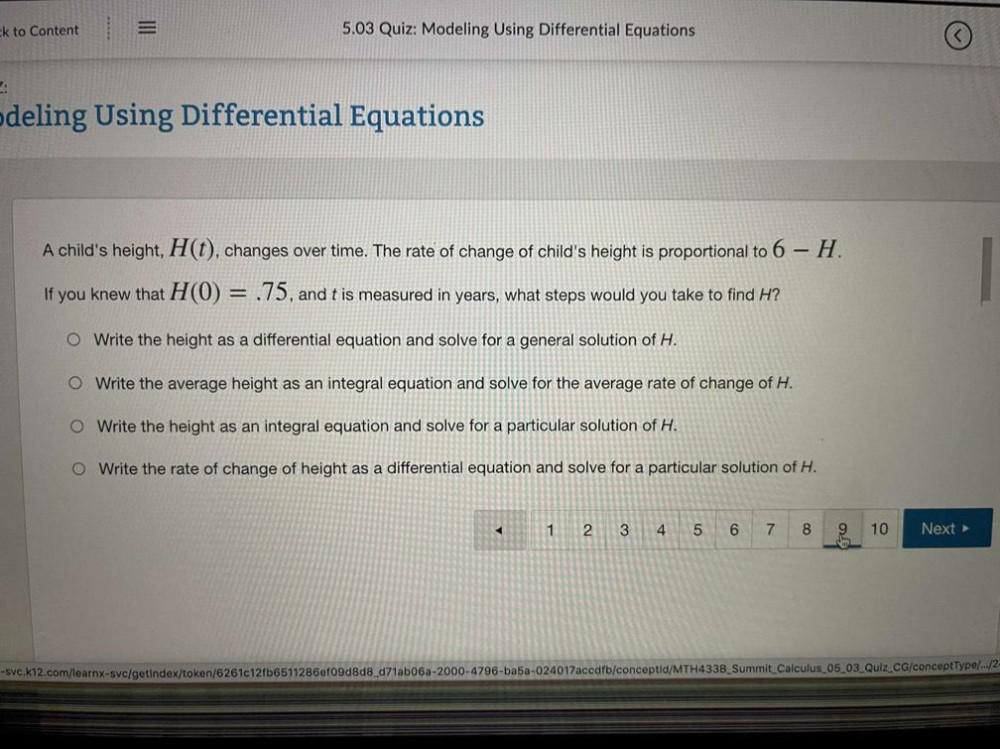Question:

# ck to Content 5.03 Quiz: Modeling Using Differential Equations deling Using Differential Equations A child's height, H(t), changck to Content 5.03 Quiz: Modeling Using Differential Equations deling Using Differential Equations A child's height, H(t), changes over time. The rate of change of child's height is proportional to 6 - H. If you knew that H(0) = .75, and t is measured in years, what steps would you take to find H? O Write the height as a differential equation and solve for a general solution of H. O Write the average height as an integral equation and solve for the average rate of change of H. O Write the height as an integral equation and solve for a particular solution of H. O Write the rate of change of height as a differential equation and solve for a particular solution of H. 1 2 3 4 5 6 7 8 9 10 Next > SVC.k12.com/learnx-svc/getindex/token/62610121b6511286ef09d8d8_d71ab05a-2000-4796-ba5a-024017accdfb/conceptid/MTH433B_Summit Calculus 05_03_Quiz CG/conceptType/../2-